# NCERT Solutions for Class 9 Maths Chapter 9 Areas of Parallelograms and Triangles

NCERT solutions for class 9 maths chapter 9 Areas of Parallelograms and Triangles: A four side shape having equal opposite sides and whose diagonals bisect each other is called a parallelogram. Triangle is a 3 side shape having 3 sides and 3 angles. In this particular chapter, you will learn about the areas of different triangles and parallelograms. Solutions of NCERT class 9 maths chapter 9 Areas of Parallelograms and Triangles will provide you the solutions to all types of triangles and parallelograms covered in the chapter. Many structures have the shape of the triangle and parallelograms. Understanding the area is mandatory in the field of calculating the area of land. If a land has an irregular shape, the land will be divided into triangles and the area will be calculated.

CBSE NCERT solutions for class 9 maths chapter 9 Areas Of Parallelograms And Triangles is also covering the solutions to the application based questions as well. There are several interesting problems in the chapter. Solving these problems will help you in improving the concepts of the chapter and also in exams like the olympiads. In total there are 4 practice exercises having 51 questions. NCERT solutions for class 9 maths chapter 9 Areas of Parallelograms and Triangles have covered all the exercises including the optional ones in a detailed manner. NCERT solutions are available for other classes and chapters as well. Four exercises of this chapter are explained below.

Exercise:9.1

Exercise:9.2

Exercise:9.3

Exercise:9.4

## NCERT solutions for class 9 maths chapter 9 Areas Of Parallelograms And Triangles Excercise: 9.1

### Q Which of the following figures lie on the same base and between the same parallels. In such a case, write the common base and the two parallels.In figure (i), (iii) and (v) we can see that. they lie on the same base and between the same parallel lines.
In figure (i)      figure (iii)     figure (v)
Two parallels         DC and AB     QR and PS    AD and BQ

## NCERT solutions for class 9 maths chapter 9 Areas Of Parallelograms And Triangles Excercise: 9.2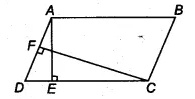We have,
AB = 16 cm, AE = 8 cm and CF = 10 cm

Since ABCD is a parallelogram,
therefore, AB = DC = 16 cm
We know that, area of parallelogram (ABCD) = base . height
= CD  AE  = (16  8 )
therefore area of parallelogram  = AD  CF = 128

Thus the required length of AD is 12.8 cm.

Join GE and HE,
Since F, F, G, H are the mid-points of the parallelogram ABCD. Therefore, GE || BC ||AD and HF || DC || AB.
It is known that if a triangle and the parallelogram are on the same base and between the same parallel lines. then the area of the triangle is equal to the half of the area of the parallelogram.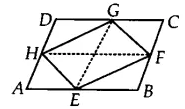Now, EFG and ||gm BEGC are on the same base and between the same parallels EG and BC.
Therefore, ar (EFG) =  ar (||gm BEGC)...............(i)
Similarly, ar (EHG) = 1/2 . ar(||gm AEGD)..................(ii)
By adding eq (i) and eq (ii), we get

ar (EFG) +  ar (EHG) = 1/2 (ar (||gm BEGC) + ar(||gm AEGD))
ar (EFGH) = 1/2 ar(ABCD)
Hence proved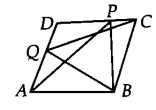We have,
ABCD is a parallelogram, therefore AB || CD and BC || AD.

Now,  APB and ||gm ABCD are on the same base AB and between two parallels AB and DC.
Therefore, ar  (APB) = 1/2 . ar(||gm ABCD)...........(i)

Also, BQC and ||gm ABCD are on the same base BC and between two parallels BC and AD.
Therefore, ar(BQC) = 1/2 . ar(||gmABCD)...........(ii)

From eq(i) and eq (ii),  we get,
ar  (APB) =  ar(BQC)
Hence proved.

[Hint : Through P, draw a line parallel to AB.]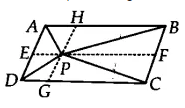We have a ||gm ABCD and AB || CD, AD || BC.  Through P, draw a line parallel to AB
Now, APB and ||gm ABEFare on the same base AB and between the same parallels EF and AB.
Therefore, ar (APB) = 1/2 . ar(ABEF)...............(i)
Similarly, ar (PCD ) = 1/2 . ar (EFDC) ..............(ii)
Now, by adding both equations, we get

Hence proved.

[Hint : Through P, draw a line parallel to AB.]We have a ||gm ABCD and AB || CD, AD || BC.  Through P, draw a line parallel to AB
Now, APD and ||gm ADGHare on the same base AD and between the same parallels GH and AD.
Therefore, ar (APD) = 1/2 . ar(||gm ADGH).............(i)

Similarily, ar (PBC) = 1/2 . ar(||gm BCGH)............(ii)

By adding the equation i and eq (ii), we get

Hence proved.

(i)
(ii)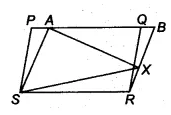(i) Parallelogram PQRS and ABRS are on the same base RS and between the same parallels RS and PB.
Therefore,  ............(i)
Hence proved

(ii) AXS and ||gm ABRS are on the same base AS and between same parallels AS and RB.
Therefore, ar (AXS) = 1/2 . ar(||gm ABRS)............(ii)

Now, from equation (i) and equation (ii), we get

Hence proved.

We have a field in the form of parallelogram PQRS and a point A is on the side RS. Join AP and AQ. The field is divided into three parts i.e, APS, QAR and PAQ.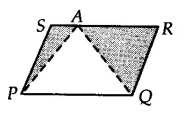Since APQ and parallelogram, PQRS is on the same base PQ and between same parallels RS and PQ.
Therefore, ............(i)
We can write above equation as,

ar (||gm PQRS) - [ar (APS) + ar(QAR)] = 1/2 .ar(PQRS)

from equation (i),

Hence, she can sow wheat in APQ and pulses in [APS + QAR] or wheat in [APS + QAR] and pulses in  APQ.

## NCERT solutions for class 9 maths chapter 9 Areas Of Parallelograms And Triangles Excercise: 9.3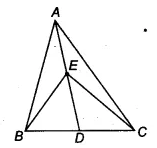We have ABC such that AD is a median. And we know that median divides the triangle into two triangles of equal areas.
Therefore, ar(ABD) = ar( ACD)............(i)

Similarly, In triangle BEC,
ar(BED) = ar (DEC)................(ii)

On subtracting eq(ii) from eq(i), we get
ar(ABD) - ar(BED) =

Hence proved.

We have a triangle ABC and  AD is a median. Join B and E.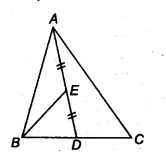Since the median divides the triangle into two triangles of equal area.
ar(ABD) = ar (ACD) = 1/2 ar(ABC)..............(i)
Now, in triangle ABD,
BE is the median [since E is the midpoint of AD]
ar (BED) = 1/2 ar(ABD)........(ii)

From eq (i) and eq (ii), we get

ar (BED) = 1/2 . (1/2 ar(ar (ABC))
ar (BED)  = 1/4 .ar(ABC)

Hence proved.Let ABCD is a parallelogram. So, AB || CD and AD || BC and we know that Diagonals bisects each other. Therefore, AO = OC and BO = OD

Since OD = BO
Therefore, ar (BOC) = ar (DOC)...........(a)   ( since OC is the median of triangle CBD)

Similarly, ar(AOD) = ar(DOC) ............(b)     ( since OD is the median of triangle ACD)

and, ar (AOB) = ar(BOC)..............(c)           ( since OB  is the median of triangle ABC)

From eq (a), (b) and eq (c), we get

ar (BOC) = ar (DOC)= ar(AOD) =  (AOB)

Thus, the diagonals of ||gm divide it into four equal triangles of equal area.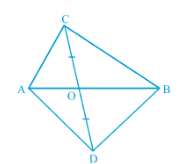We have, ABC and ABD on the same base AB. CD is bisected by AB at point O.
OC = OD
Now, in ACD, AO is median
ar (AOC) = ar (AOD)..........(i)

Similarly, in BCD, BO is the median
ar (BOC) = ar (BOD)............(ii)

Adding equation (i) and eq (ii), we get

ar (AOC) + ar (BOC) =  ar (AOD) + ar (BOD)

Hence proved.

We have a triangle ABC such that D, E and F are the midpoints of the sides BC, CA and AB respectively.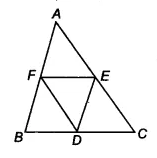Now, in ABC,
F and E are the midpoints of the side AB and AC.
Therefore according to mid-point theorem, the line segment connecting the midpoints of two sides of a triangle is parallel to the third side and half of the third side.
EF || BC or EF || BD

also, EF = 1/2 (BC)
[ D is the midpoint of BC]
Similarly, ED || BF and ED = FB
Hence BDEF is a parallelogram.We already proved that BDEF is a ||gm.
Similarly, DCEF and DEAF are also parallelograms.
Now, ||gm BDEF and ||gm DCEF is on the same base EF and between same parallels BC and EF
Ar (BDEF) = Ar (DCEF)
Ar(BDF) = Ar (DEF) .............(i)

It is known that diagonals of ||gm divides it into two triangles of equal area.
Ar(DCE) = Ar (DEF).......(ii)

and, Ar(AEF) = Ar (DEF)...........(iii)

From equation(i), (ii) and (iii), we get

Ar(BDF) =  Ar(DCE) = Ar(AEF) =  Ar (DEF)

Thus, Ar (ABC) =  Ar(BDF) +  Ar(DCE) + Ar(AEF) +  Ar (DEF)
Ar (ABC) = 4 . Ar(DEF)

Hence proved.ar(DEF) = ar ( BDF).........(i)

So, ar(||gm BDEF) = ar(BDF) + ar (DEF)
= 2 . ar(DEF)    [from equation (i)]

Hence proved.

[Hint: From D and B, draw perpendiculars to AC.]We have ABCD is quadrilateral whose diagonals AC and BD intersect at O. And OB = OD, AB = CD
Draw DE  AC and FB AC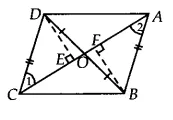In DEO and  BFO
DOE = BOF [vertically opposite angle]
OED = BFO [each ]
OB = OD [given]

Therefore, by AAS congruency
DEO    BFO
DE = FB [by CPCT]

and ar( DEO) =  ar(BFO) ............(i)

Now, In  DEC and  ABF
DEC = BFA [ each ]
DE = FB
DC = BA [given]
So, by RHS congruency
DEC    BFA
1 = 2 [by CPCT]
and, ar( DEC) =  ar(BFA).....(ii)

By adding equation(i) and (ii), we get

Hence proved.

[Hint: From D and B, draw perpendiculars to AC.]Now, add  on both sides we get

Hence proved.

or ABCD is a parallelogram.

[Hint : From D and B, draw perpendiculars to AC.]Since DCB and ACB both lie on the same base BC and having equal areas.
Therefore, They lie between the same parallels BC and AD
also 1 = 2 [ already proved]
So, AB ||  CD
Hence ABCD is a || gm

We have   ABC and points D and E are on the sides AB and AC such that ar(DBC ) = ar (EBC)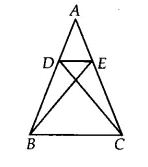Since DBC and EBC are on the same base BC and having the same area.
They must lie between the same parallels DE and BC
Hence DE || BC.

We have a ABC  such that BE || AC and CF || AB
Since XY || BC and BE || CY
Therefore, BCYE is a ||gmNow, The ||gm BCEY and ABE are on the same base BE and between the same parallels AC and BE.
ar(AEB) = 1/2 .ar(||gm BEYC)..........(i)
Similarly, ar(ACF) = 1/2 . ar(||gm BCFX)..................(ii)

Also, ||gm BEYC and ||gmBCFX are on the same base BC and between the same parallels BC and EF.
ar (BEYC) = ar (BCFX).........(iii)

From eq (i), (ii) and (iii),  we get

ar( ABE) = ar(ACF)
Hence proved.Join the AC and PQ.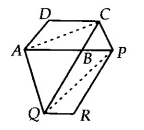It is given that ABCD is a ||gm and AC is a diagonal of ||gm
Therefore, ar(ABC) = ar(ADC) = 1/2 ar(||gm ABCD).............(i)

Also, ar(PQR) = ar(BPQ) = 1/2 ar(||gm PBQR).............(ii)

Since AQC and APQ are on the same base AQ and between same parallels AQ and CP.
ar(AQC) = ar (APQ)

Now, subtracting ABQ from both sides we get,

ar(AQC) - ar (ABQ) = ar (APQ) - ar (ABQ)
ar(ABC) = ar (BPQ)............(iii)

From eq(i), (ii) and (iii) we get

Hence proved.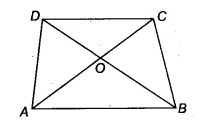We have a trapezium ABCD such that AB || CD and it's diagonals AC and BD intersect each other at O
ABD and  ABC are on the same base AB and between same parallels AB and CD
ar(ABD) = ar (ABC)

Now, subtracting AOB from both sides we get

ar ( AOD) = ar ( BOC)

Hence proved.

(i)
(ii)